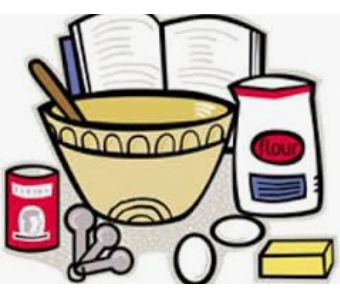Culminating Semester Project (AP Cal AB / BC)Calculus Recipe Project

The purpose of this project is to rewrite a recipe using elements of calculus.

Part 1 (25 points): The student must choose a recipe that contains a minimum of 10 numerical values in a recipe. This values include ingredients amount, cooking temperature, baking or cooking time, serving size, etc.

Part 2 (25 points): The student must rewrite all numerical values using the following types of problems one time each. AP Calculus BC students must use at most five AB topics and the rest of the recipe must be written using BC topics
.

• Limits using factoring, rationalizing the numerator, or least common denominator
• Limits at Infinity
• Limit using L'hopital's Rule
• Slope of a tangent line using the Power Rule
• Slope of a tangent line using the Product Rule
• Slope of a tangent line using the Quotient Rule
• Slope of a tangent line using the Chain Rule
• Slope of a tangent line using Implicit Differentiation
• Relative minimum or maximum value
• Absolute minimum or maximum value
• Inflection point
• Left Riemann Sum
• Right Riemann Sum
• Definite integral using FTC
• Definite integral using u-substitution
• Definite integral using integration by parts (BC only)
• Definite integral using partial fractions (BC only)
• Improper integral that converges (BC only)
• Convergent geometric series (BC only)
• Radius of convergence of a power series (BC only)
• Arc length in rectangular form (BC only)
• Speed of a particle using parametric equations (BC only)
Part 3 (50 points): Students must submit a PowerPoint or Prezi with the following slides:
• Slide 1: Title Slide - Name, Date, Period / Day
• Slide 2: Original recipe
• Slide 3: Rewritten recipe using calculus topics using proper notation
• Slide 4 and beyond: Work shown for each ingredient of the recipe using proper notation and justifying answers when necessary